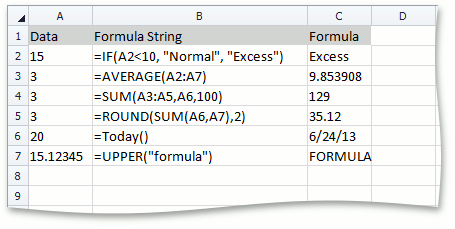21.2 (EAP/Beta)
21.1
20.2
20.1
19.2
19.1
18.2
18.1
17.2

# How to: Use Functions and Nested Functions in Formulas

This example demonstrates how to use predefined functions in formulas to perform simple or complex calculations over arguments. Set the CellRange.Formula property to a formula. Follow the rules below to create a formula that uses a function.

1. Start a formula with the “=” sign, as you usually do when creating a formula.
2. Type the function name.
3. Type the function arguments in parentheses. Arguments can be numbers, text and logical values, cell references and names, or other functions.

``````// If the number in cell A2 is less than 10, the formula returns "Normal"
// and this text is displayed in cell C2. Otherwise, cell C2 displays "Excess".
worksheet.Cells["C2"].Formula = @"=IF(A2<10, ""Normal"", ""Excess"")";

// Calculate the average value for cell values within the "A2:A7" range.
worksheet.Cells["C3"].Formula = "=AVERAGE(A2:A7)";

// Add the values contained in cells A3 through A5, add the value contained in cell A6,
// and add 100 to that result.
worksheet.Cells["C4"].Formula = "=SUM(A3:A5,A6,100)";

// Use a nested function in a formula.
// Round the sum of the values contained in cells A6 and A7 to two decimal places.
worksheet.Cells["C5"].Formula = "=ROUND(SUM(A6,A7),2)";

// Add the current date to cell C6.
worksheet.Cells["C6"].Formula = "=Today()";
worksheet.Cells["C6"].NumberFormat = "m/d/yy";

// Convert the specified text to uppercase.
worksheet.Cells["C7"].Formula = @"=UPPER(""formula"")";
``````

The image below shows cells with formulas using different functions.